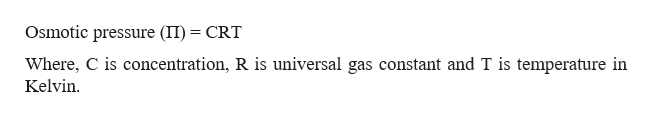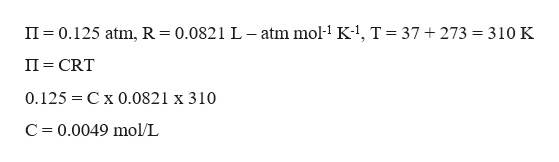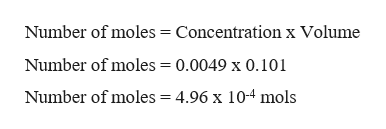# When 2.63 g of a polypeptide is dissolved in 101 mL of water, the resulting solution is found to have an osmotic pressure of 0.125 atm at 37.0 °C. What is the molar mass of the polypeptide? (Assume the volume doesn't change when the polypeptide is added.)

Question
6 views

When 2.63 g of a polypeptide is dissolved in 101 mL of water, the resulting solution is found to have an osmotic pressure of 0.125 atm at 37.0 °C. What is the molar mass of the polypeptide? (Assume the volume doesn't change when the polypeptide is added.)

check_circle

Step 1

To calculate the molecular weight first it required to calculate the number of moles and to calculate number of moles, it is required to calculate the concentration of the substance which can be calculated as,help_outlineImage TranscriptioncloseOsmotic pressure (II) CRT Where, C is concentration, R is universal gas constant and T is temperature in Kelvin fullscreen
Step 2

Substituting the values to calculate the concentration.help_outlineImage TranscriptioncloseII 0.125 atm, R 0.0821 L-atm mol1 K- T 37 + 273 = 310 K II CRT 0.125 C x 0.0821 x 310 C 0.0049 mol/L fullscreen
Step 3

Now since the volume is given as 101 mL (0.101 L...help_outlineImage TranscriptioncloseNumber of moles = Concentration x Volume Number of moles = 0.0049 x 0.101 Number of moles = 4.96 x 104 mols fullscreen

### Want to see the full answer?

See Solution

#### Want to see this answer and more?

Solutions are written by subject experts who are available 24/7. Questions are typically answered within 1 hour.*

See Solution
*Response times may vary by subject and question.
Tagged in

### Solutions